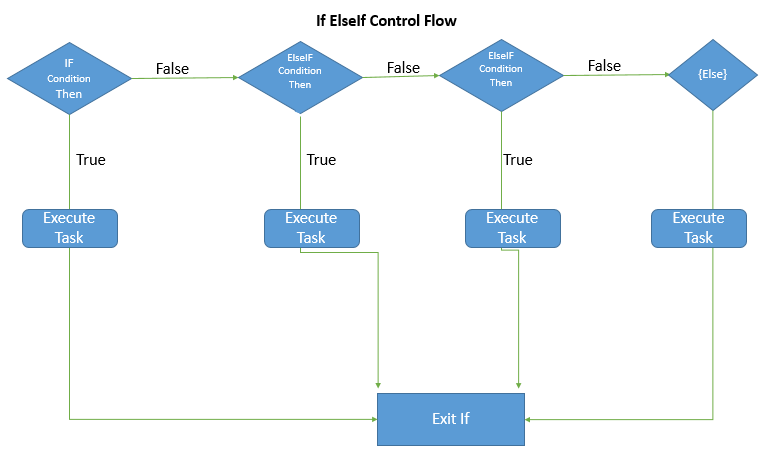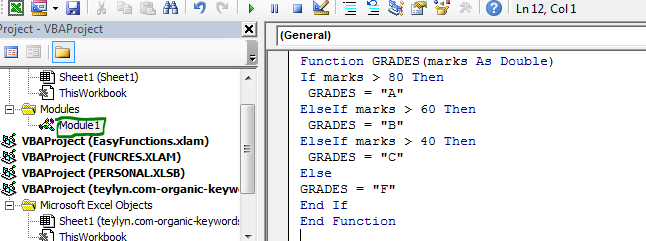# The If ElseIf Statement in VBA

There will be times when you want to do different tasks depending on different conditions. You would like to check different conditions if one condition falls. In that scenario we use the If ElseIf statements in VBA.

Syntax of If ElseIf in VBA

```If condition1 then
'Code to execute if condition1 is true
ElseIF Condition2 then
'Code to execute if condition2 is true
ElseIF Condition3 then
'Code to execute if condition3 is true
'--
'--
ElseIF ConditionN then
'Code to execute if conditionN is true

{Else}
'Optional Code if none of the condition is matched.

End If Sub
```

Control Flow in If ElseIf StatementsIn If ElseIf statement, the next condition is only checked when the previous condition falls. When a condition is matched, the code in that block is executed and the control exits the If block. There's one Else block that is optional. It is only executed if none of the conditions are matched. If you want to do something if none of the conditions are matched then put that code in the Else block.

So, enough of the theories. Let's have an example to digest it.
Example: Grade Marks Using VBA If ElseIf Statements
Here we are taking the classic example of the grading system. We want to create a user defined function GRADE that grades the marks according to below conditions:
If marks are greater than 80, grade A. Else, if marks are greater than 60, grade B. Else, if marks are greater than or equal to 40, grade C. If marks are less than 40, grade F.

Here's the VBA code:

```Function GRADES(marks As Double)
If marks > 80 Then
ElseIf marks > 60 Then
ElseIf marks > 40 Then
Else
End If
End Function
```

The above function first checks if the supplied value is greater than 80. If this condition falls True, the function returns A and exits the if block. If the condition is not matched then it checks the next ElseIF condition. If none of the conditions are True then Else block is executed and Grade F is returned.If ElseIf Vs Nested Ifs
If Else If is not nested Ifs, as nested Ifs checks another condition when previous condition was matched. Where is If ElseIf statement checks another condition when previous condition is not matched.

In If ElseIf, when one condition is matched, the code in that condition is executed and control exits the If ElseIf statements. Where in nested Ifs, when one condition is matched another condition is checked. The control exits when any condition is False or all conditions are matched.

So yeah guys, this is If ElseIF statements in VBA. I hope this was useful to you. Let me know if this was explanatory enough in the comments section below. If you have any doubts or special requirements in VBA or Excel Formulas, ask in the comments section below.

Related Articles:

VBA Select Case Statement: The Select Case Statements are useful when you have too many conditions to check. They are excellent replacements of multiple If ElseIf statements.

Using Loop in VBA in Microsoft Excel | The loops in VBA enable us to do a similar task over and over without repetition of code. There are 3 types of loops in Excel VBA.

7 Examples of For Loops in Microsoft Excel VBA | The 7 examples of for loop can make your automation life easy. Let's start with simple for loop.

Popular Articles:

50 Excel Shortcuts to Increase Your Productivity | Get faster at your task. These 50 shortcuts will make you work even faster on Excel.

The VLOOKUP Function in Excel | This is one of the most used and popular functions of excel that is used to lookup value from different ranges and sheets.

COUNTIF in Excel 2016 | Count values with conditions using this amazing function. You don't need to filter your data to count specific values. Countif function is essential to prepare your dashboard.

How to Use SUMIF Function in Excel | This is another dashboard essential function. This helps you sum up values on specific conditions.

Terms and Conditions of use

The applications/code on this site are distributed as is and without warranties or liability. In no event shall the owner of the copyrights, or the authors of the applications/code be liable for any loss of profit, any problems or any damage resulting from the use or evaluation of the applications/code.